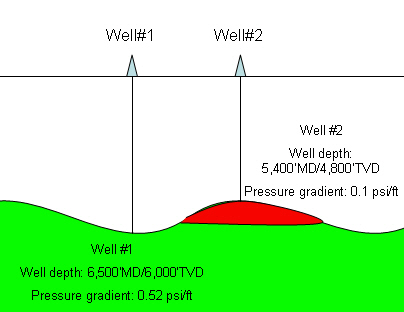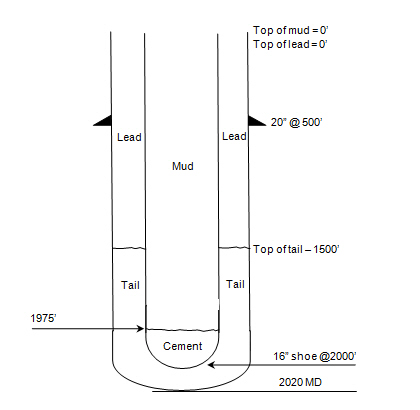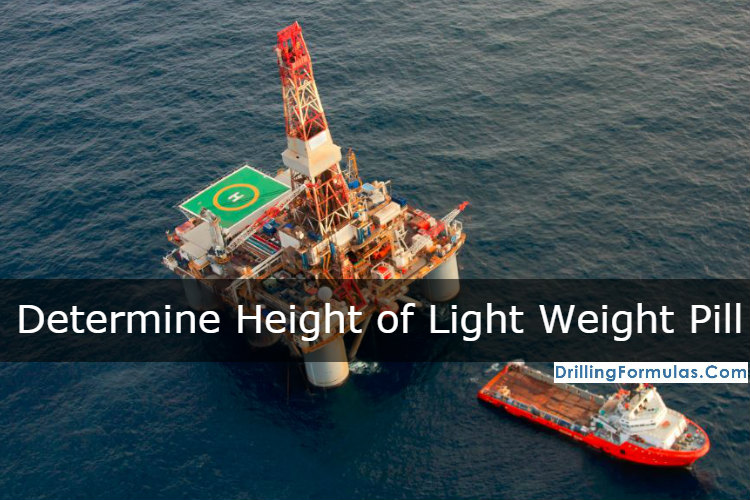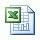## Abnormal Pressure from Anticline Gas Cap

I got a question about how an anticline gas cap can create the abnormal pressure. The anticline with gas cap can be the potential high pressurized zone. Because of reservoir connectivity between fluid underneath gas and gas reservoir, it can generate abnormal pressure. This example below demonstrates you how this situation could happen.## How much pressure will we see at cement head in case of float shoe fail?

I still have the simple but interesting question about hydrostatic pressure which you can apply this calculation into drilling/cementing operation. The question I got about how much pressure we will see at cement head in case of float shoe fail.## Mixing Fluids of Different Densities with Pit Space Limitation

You many have different drilling fluid densities in your mud pits so you can have option to mix different fluid densities together in order to get you desired mud weight and desired volume. The concept is to weighted average volume and density of each mud component. The calculation is show as a formula below

(V1 x D1) + (V2 x D2) = VF x DF

Where;

V1 = volume of fluid 1 (bbl, gal, etc.)

D1 = density of fluid 1 (ppg,lb/ft3, etc.)

V2 = volume of fluid 2 (bbl, gal, etc.)

D2 = density of fluid 2 (ppg,lb/ft3, etc.)

VF = volume of final fluid mix

DF = density of final fluid mix

There are 2 cases of calculations as follows:

Case#1: You have limitation of pit space.

Case#2: You don’t have limitation of pit space.

Let’s read and understand how to calculate each case.

Case#1: You have limitation of pit space.

This case you can not apply only formula as show above but you need to add one formula and then you use relationship between 2 equations to sovle your problem.

Determine the volume of 10.0 ppg mud and 14.0 ppg mud required to build 300 bbl of

12.0 ppg mud:

You have following mud in you mud pit.

• 300 bbl of 10.0 ppg mud in pit A

• 300 bbl of 14.0 ppg mud in pitB

Solution:

V1 = volume of 10.0 ppg mud required to mix

V2 = volume of 14.0 ppg mud required to mix

So total volume required: V1 + V2 = 300 bbl -> Equation#1

Apply formula of mixing different fluid density.

(V1 x D1) + (V2 x D2) = VF x DF

Where;

V1 = volume of 10.0 ppg mud required to mix

D1 = 10 ppg

V2 = volume of 14.0 ppg mud required to mix

D2 = 14.0 ppg

VF = 300 bbl

DF = 12.0 ppg

(V1 x10.0) + (V2 x 14.0) = 300 x 12.0 -> Equation#2

From Equation#1: V1 = 300 – V2.

Use this relationship to Equation#2

((300-V2) x 10.0) + (V2 x 14.0) = 3,600

3000 – 10V2 + 14V2 = 3600

4V2 = 600

V2 = 150

So volume of 14.0 ppg mud required to mix = 150 bbl

Let’s go back to Equation#1 and put V2 that you just solve so you will get V1

V1 + V2 = 300 bbl

V1 + 150 = 300

V1 = 150 bbl

In order to get 300 bbl of 12.0 ppg mud, you need to mix 150 bbl of 10.0 ppg with 150 bbl of 14.0 ppg.

The case#2 will be posted on the next post so please continue in next few days.

Please find the excel sheet to calculate “mix different mud density with limited pit space”

## Determine height of light weight spot pill to balance formation pressureWhen you get differentially stuck, you may consider spotting light weight fluid to reduce force created by differential pressure between mud in wellbore and formation pressure. However, it is imperative to be able to calculate how much light weight fluid added into the well that it will not  accidentally underbalance formation pressure.Please follow the steps below to determine how height of light weight spot pill in the annulus to balance formation pressure.

a) Determine the difference in pressure gradient in psi/ft between the mud weight and light weight spot fluid:

Difference pressure in pressure gradient in psi/ft = (current mud wt in ppg – light weight spot pill in ppg) x 0.052

b) Determine height in ft of light weight spot fluid that will balance formation pressure in the annulus:

Height ft in vertical = overbalance pressure with current mud weigh in psi ÷ difference in pressure gradient in psi/ft

This height is the maximum allowable height of light weight spot pill in the annulus. If you have higher length of light weight spot pill in the annulus, it may create a well control situation.

Example: Use the following data to determine the height in ft of light weight spot pill that will balance formation pressure in the annulus:

Mud weight = 13.0 ppg

Light weight spot pill = 8.3 ppg

Amount of overbalance = 300 psi

a) Difference in pressure gradient in psi/ft:

Difference pressure in pressure gradient in psi/ft = (13 ppg – 8.3 ppg) x 0.052

Difference pressure in pressure gradient = 0.2444 psi/ft

b) Determine the height in ft of light weight spot liquid that will balance formation pressure in the annulus:

Height = 300 psi ÷ 0.244 psi/ft

Height = 1227 ft

You must ensure that height of light weight pill in the annulus must be less than 1227 ft in order to prevent a wellcontrol situation.

In order to determine light weight pill volume, this simple formula below is used;

volume of pill = annular capacity (bbl/ft) x height of pill (ft)

For instant, the annular capacity is 0.0459 bbl/ft, volume of pill is equal to 56.3 bbl (1,227 x 0.0459).

Ref books: Lapeyrouse, N.J., 2002. Formulas and calculations for drilling, production and workover, Boston: Gulf Professional publishing.

Bourgoyne, A.J.T., Chenevert , M.E. & Millheim, K.K., 1986. SPE Textbook Series, Volume 2: Applied Drilling Engineering, Society of Petroleum Engineers.

Mitchell, R.F., Miska, S. & Aadny, B.S., 2011. Fundamentals of drilling engineering, Richardson, TX: Society of Petroleum Engineers.

## Amount of cuttings produced per foot of hole and total solid generatedAfter learning about capacity calculation, we can apply the capacity calculation to determine how much barrels of cutting produced per foot of hole drilled and total solid generated in pounds.

Please read and understand the following formulas:

Use formula#1 and #2 for calculating amount of cutting generated per feet drilled.

## Formula#1 for BARRELS of cuttings drilled per foot of hole drilled:

Barrels of cutting per foot drilled = Dh2 x (1 – % porosity) ÷1029.4

Where: Dh is hole diameter in inch.

Example: Determine barrels of cuttings drilled for one foot of 6-1/8 inch hole with 25% (0.25) porosity:

Barrels/footage drilled = 6.1252 x (1 – 0.25) ÷1029.4
Barrels/footage drilled = 0.02733 bbl/footage drilled

## Formula#2 for CUBIC FEET of cuttings drilled per foot of hole drilled:

Cubic feet of cutting per foot drilled = Dh2 x 0.7854 x (1 – % porosity) ÷144

Where: Dh is hole diameter in inch.

Example: Determine barrels of cuttings drilled for one foot of 6-1/8 inch hole with 25% (0.25) porosity

Cubic feet/footage drilled = 6.1252 x 0.7854 x (1 – 0.25) ÷144
Cubic feet/footage drilled = 0.153462 cu ft/footage drilled

Moreover, you also apply sample density and volume relationship to determine total solids generated. Use the following formula to calculate total solid generated.

Wcg = 350 x Ch x L x (l – porosity) x Cutting density

Where;

Wcg = solids generated in pounds
Ch = capacity of hole in bbl/ft
L = footage drilled in ft
Cutting density = cutting density in gm/cc

Example: Determine the total pounds of solids generated in drilling 100 ft of  6-1/8 inch hole (0.03644 bbl/ft).

Density of cuttings = 2.20 gm/cc.

Porosity = 25%:
Wcg = 350 x 0.03644 x 100 x (1 – 0.25) x 2.2
Wcg = 2104.41 poundsPlease find the excel sheet how to calculate how much cuttings drilled per foot of hole drilled and total solids generated

Ref books: Lapeyrouse, N.J., 2002. Formulas and calculations for drilling, production and workover, Boston: Gulf Professional publishing.

Bourgoyne, A.J.T., Chenevert , M.E. & Millheim, K.K., 1986. SPE Textbook Series, Volume 2: Applied Drilling Engineering, Society of Petroleum Engineers.

Mitchell, R.F., Miska, S. & Aadny, B.S., 2011. Fundamentals of drilling engineering, Richardson, TX: Society of Petroleum Engineers.